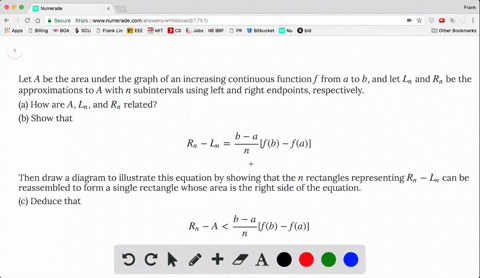### Let $A$ be the area under the graph of an incre…

03:42
FL
Problem 26

(a) Use Definition 2 to find an expression for the area under the curve $y = x^3$ from 0 to 1 as a limit.

(b) The following formula for the sum of the cubes of the first $n$ integers is proved in Appendix E. Use it to evaluate the limit in part (a).

$$1^3 + 2^3 + 3^3 + \cdots + n^3 = \biggl[ \frac{n(n + 1)}{2} \biggr]^2$$

(A). $\lim _{n \rightarrow \infty} \sum_{i=1}^{n} \frac{i^{3}}{n^{4}}$
(B). $\frac{1}{4}$

## Discussion

You must be signed in to discuss.

## Video Transcript

So try to figure out this inter crow. And I mean, we right out of general formula for this specific proper and are a zero piece one and the F of axis x Q. So this gives us one of run some eye from one, two and eight zero piece one. So this high over on Q so high to over on cue and by the formula given impart, be so this. So this will be answered ofthe party and by this given form last. We know that this you can compare the leave me like this We fact at once or over a cube. So we are one over on to the fourth and we some over I too, from one toe on which it's given by this formula. So is for on square on plus one square. And this limits one over for people's we have for Lamia enumeration denominator. They have the same decree degree course for so the limit is just a racial off coefficient of the highest order term in this case will be one over four